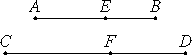# Proposition 68

A straight line commensurable with a major straight line is itself also major.

Let AB be major, and let CD be commensurable with AB.

I say that CD is major.X.39

Divide AB at E. Then AE and EB are straight lines incommensurable in square which make the sum of the squares on them rational but the rectangle contained by them medial.

Make the same construction as before.

V.11

Since AB is to CD as AE is to CF, and EB is to FD, therefore AE is to CF as EB is to FD.

X.11

But AB is commensurable with CD, therefore AE and EB are commensurable with CF and FD respectively.

Since AE is to CF as EB is to FD, alternately, also AE is to EB as CF is to FD, therefore, taken jointly, AB is to BE as CD is to DF.

VI.20

Therefore the square on AB is to the square on BE as the square on CD is to the square on DF.

V.16

Similarly we can prove that the square on AB is to the square on AE as the square on CD is to the square on CF. Therefore the square on AB is to the squares on AE and EB as the square on CD is to the squares on CF and FD, therefore, alternately, the square on AB is to the square on CD, so are the squares on AE and EB to the squares on CF and FD.

But the square on AB is commensurable with the square on CD, therefore the squares on AE and EB are also commensurable with the squares on CF and FD.

And the squares on AE and EB together are rational, therefore the squares on CF and FD together are rational.

Similarly also twice the rectangle AE by EB is commensurable with twice the rectangle CF by FD.

X.23,Cor.

And twice the rectangle AE by EB is medial, therefore twice the rectangle CF by FD is also medial.

X.39

Therefore CF and FD are straight lines incommensurable in square which make, at the same time, the sum of the squares on them rational, but the rectangle contained by them medial, therefore the whole CD is the irrational straight line called major.

Therefore a straight line commensurable with the major straight line is major.

Therefore, a straight line commensurable with a major straight line is itself also major.

Q.E.D.

## Guide

This proposition is not used in the rest of the Elements